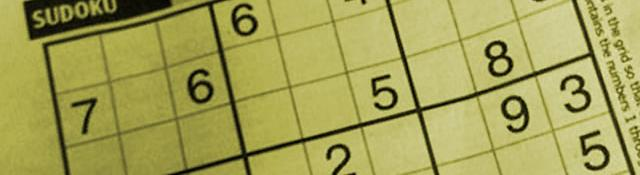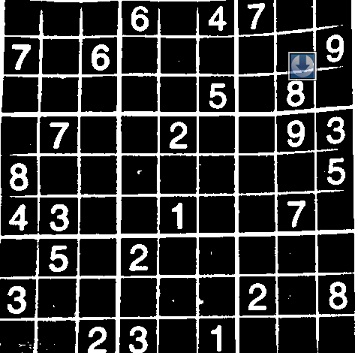## Recognizing digits

Series: SuDoKu Grabber in OpenCV:

1. The Plot
2. Grid detection
3. Extracting the grid
4. Extracting digits
5. Recognizing digits

In the previous article (Detecting a SuDoKu puzzle in an Image, Part 3), we created the DigitRecognizer class.It had functions for training and classifying images as digits. We also put some pre-processing code to ensure the image is at the center. Now, we'll combine it with our existing program. We'll also use the merged lines we created earlier.

## Training the classifier

We'll start where we left the original SuDoKu program. Your last line was probably:

```cv::warpPerspective(original, undistorted, cv::getPerspectiveTransform(src, dst), Size(maxLength, maxLength));
```

Unless you tried hacking a bit yourself!

First thing we'll do is create a thresholded image. Till now, the text is in black. But we want it to be white. So, we'll create a duplicate and use an inverted threshold:

```Mat undistortedThreshed = undistorted.clone();
adaptiveThreshold(undistorted, undistortedThreshed, 255, CV_ADAPTIVE_THRESH_GAUSSIAN_C, CV_THRESH_BINARY_INV, 101, 1);
```

I've used the adaptive threshold (with a block size of 101 to calculate the dynamic threshold value) to keep the effects of lighting from spoiling the thresholding.

Had I used standard thresholding, it would look something like this:With the adaptive thresholding, the thresholded image looks like this:Next, we create an instance of the DigitRecognizer class and train it:

```DigitRecognizer *dr = new DigitRecognizer();
bool b = dr->train("D:/Test/Character Recognition/train-images.idx3-ubyte", "D:/Test/Character Recognition/train-labels.idx1-ubyte");
```

If you want you can put a check on 'b'. If true, the training was successful, otherwise its false.

Then, we create a small image that will hold one SuDoKu cell:

```int dist = ceil((double)maxLength/9);
Mat currentCell = Mat(dist, dist, CV_8UC1);
```

Now, we iterate through each cell:

```for(int j=0;j<9;j++)
{
for(int i=0;i<9;i++)
{
```

Each of these cells correspond to certain pixels. We copy these pixels into currentCell:

```        for(int y=0;y<dist && j*dist+y<undistortedThreshed.cols;y++)
{

uchar* ptr = currentCell.ptr(y);

for(int x=0;x<dist && i*dist+x<undistortedThreshed.rows;x++)
{
ptr[x] = undistortedThreshed.at<uchar>(j*dist+y, i*dist+x);
}
}
```

Once we've copied the pixels, we calculate the number of white pixels on it. If the number is less, it's probably a blank cell. Otherwise, it's probably a numbered cell.

```        Moments m = cv::moments(currentCell, true);
int area = m.m00;
if(area > currentCell.rows*currentCell.cols/5)
{
int number = dr->classify(currentCell);
printf("%d ", number);
}
else
{
printf("  ");
}
```

And finally, we end the loops:

```    }
printf(" ");
}
```

Try running the program. You should see the SuDoKu printed on the console.

## Accuracy

Well. the recognition isn't very accurate. I can think of two main factors:

• The training data is for handwritten text
• The algorithm used isn't great

Other possible things you can use for recognizing the text are neural networks, haar networks or some chain-codes thing (the text on the SuDoKu grid has proper and well defined boundaries).

## Summary

Today, we loaded the DigitRecognizer class into the main program and made it recognize digits. And with that, the series comes to an end! Hope you've learned a lot over the course of this series.

## More in the series

This tutorial is part of a series called SuDoKu Grabber in OpenCV:

1. The Plot
2. Grid detection
3. Extracting the grid
4. Extracting digits
5. Recognizing digits

Utkarsh Sinha created AI Shack in 2010 and has since been working on computer vision and related fields. He is currently at Microsoft working on computer vision.